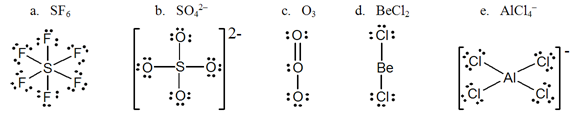# Problem: In which one of the following structures, as drawn, does the central atom have a formal charge of +2? In which one of the following structures, as drawn, does the central atom have a formal charge of +1? Answer these in order.a) c, db) a, bc) d, ed) b, ce) b, d

###### FREE Expert Solution

The formal charge can be calculated as:

**valence electron = Group number

a. SF6

FC (S) = 6 - (6 -0) = 0

81% (75 ratings)###### Problem Details

In which one of the following structures, as drawn, does the central atom have a formal charge of +2? In which one of the following structures, as drawn, does the central atom have a formal charge of +1? Answer these in order.a) c, d

b) a, b

c) d, e

d) b, c

e) b, d